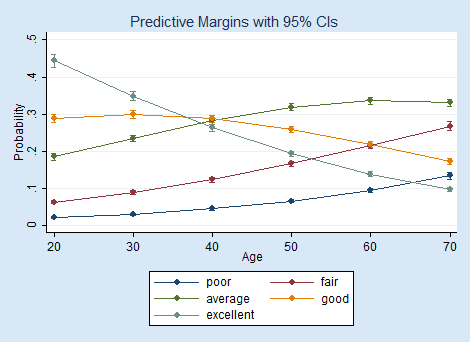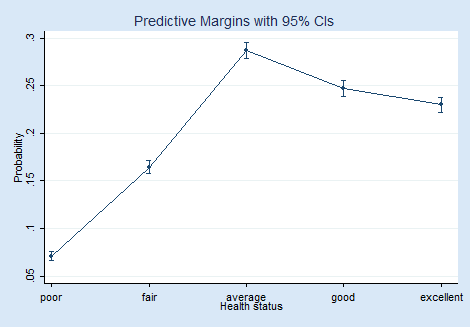Home  /  Products  /  Stata 14  /  Marginal analysis with multiple outcomes

#### Marginal analysis with multiple outcomes were introduced in Stata 14. See the latest version of marginal analysis with multiple outcomes. See the new features in Stata 18.# Marginal analysis with multiple outcomes

## Highlights

• Results automatically include all equations and outcomes
• Ordinal outcome models
• Multivariate models
• Categorical outcome models
• Automated graphs from marginsplot plot multiple outcomes too×When we fit models such as multivariate regression, MANOVA, or seemingly unrelated regression, we can make predictions for each response variable. When we fit models for ordinal or categorical response variables, we can make predictions for each outcome. margins calculates statistics such as marginal means, marginal effects, adjusted predictions, and predictive margins. These can also be computed for each response variable or for each outcome of an ordinal or a categorical variable. margins now automatically performs the requested marginal analysis for all variables and all outcomes. You can, of course, still request that it produce the analysis for only a single outcome or set of specified outcomes.

## Let's see it work

We have individuals assigned to one of five health statuses.

. tab health

1=poor,...,
5=excellent       Freq.     Percent        Cum.

poor         729        7.05        7.05
fair       1,670       16.16       23.21
average       2,938       28.43       51.64
good       2,591       25.07       76.71
excellent       2,407       23.29      100.00

Total      10,335      100.00

We fit an ordered logistic regression model for health status based on sex, age, and their interaction by typing

. ologit health i.female##c.age

We can compute the predictive margin, the average predicted probability, for each health status by simply typing margins



Delta-method
Margin   Std. Err.      z    P>|z|     [95% Conf. Interval]

_predict
1      .070893   .0024967    28.39   0.000     .0659995    .0757865
2     .1646632   .0035877    45.90   0.000     .1576315    .1716949
3     .2873167   .0044043    65.24   0.000     .2786844     .295949
4     .2469508     .00418    59.08   0.000     .2387581    .2551435
5     .2301763   .0039308    58.56   0.000      .222472    .2378806


The probability of being categorized as having average health (health=3) is higher than for any other category.

We can graph this by simply using marginsplot.How do the probabilities of being classified into each health status change with age? We compute the predictive margins for each outcome for ages ranging from 20 to 70 by typing

. margins, at(age=(20(10)70))

(output omitted)

We will not show you the table of all thirty predictive margins. It is easier to see the interaction between health status and age by using marginsplot to graph them.Based on our model, a 20-year-old is more likely to be classified as having excellent health than as having any other health status. However, a 70-year-old is most likely to be classified as having average health and least likely to be classified as having excellent health.

## Tell me more

Read more about margins, marginsplot, and all their capabilities in the Stata reference manuals. See [U] 20.15 Obtaining marginal means, adjusted predictions, and predictive margins, [R] margins, and [R] marginsplot.